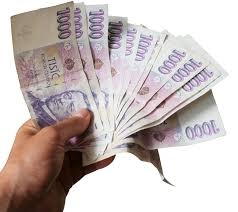# Banknotes 23691

Dad paid for the computer with banknotes worth 500 and 1,000 CZK. The number of banknotes was 56, and the price of the computer was CZK 44,000. How many banknotes did he have?

a =  24
b =  32

### Step-by-step explanation:

a+b=56
500a+1000b=44000

a+b=56
500·a+1000·b=44000

a+b = 56
500a+1000b = 44000

Pivot: Row 1 ↔ Row 2
500a+1000b = 44000
a+b = 56

Row 2 - 1/500 · Row 1 → Row 2
500a+1000b = 44000
-b = -32

b = -32/-1 = 32
a = 44000-1000b/500 = 44000-1000 · 32/500 = 24

a = 24
b = 32

Our linear equations calculator calculates it.Did you find an error or inaccuracy? Feel free to write us. Thank you!

Tips for related online calculators
Do you have a system of equations and looking for calculator system of linear equations?